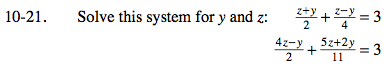### Home > A2C > Chapter Ch10 > Lesson 10.1.1 > Problem10-21

10-21.Eliminate the denominator in both equations and simplify them before solving.

$\left(\frac{z +y}{2}+\frac{z - y}{4} = 3\right)4$

$\left(\frac{4z-y}{2} + \frac{5z+2y}{11} = 3\right)22$

2z + 2y + zy = 12

44z − 11y + 10z + 4y = 66

3z + y = 12

54z − 7y = 66